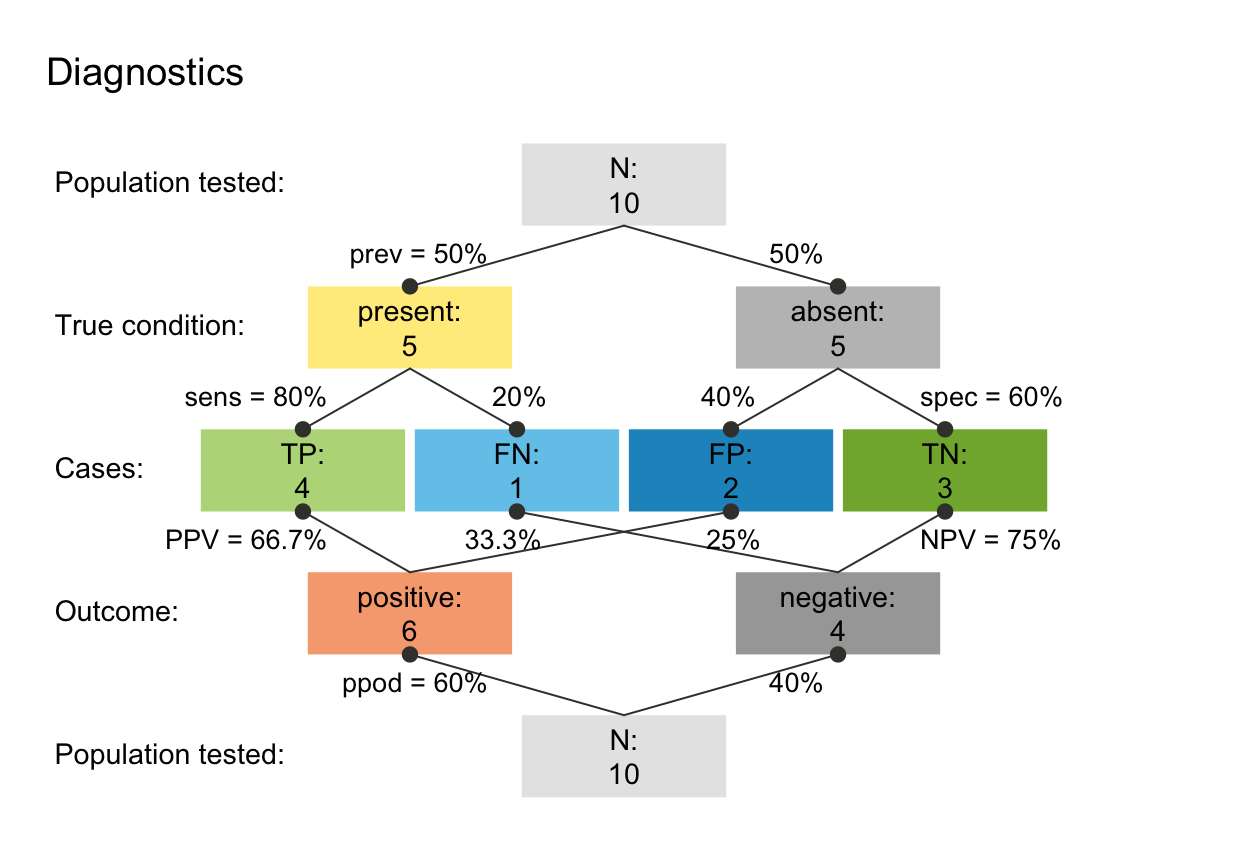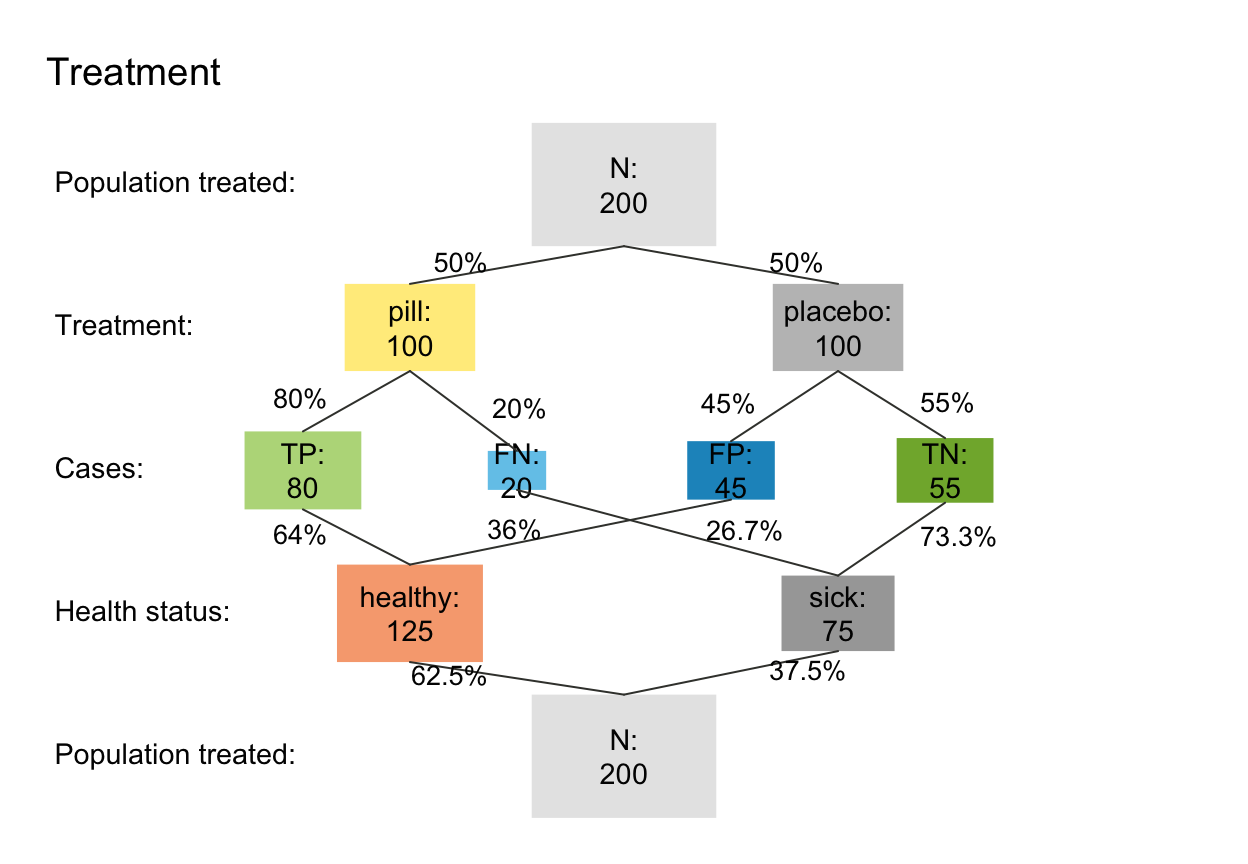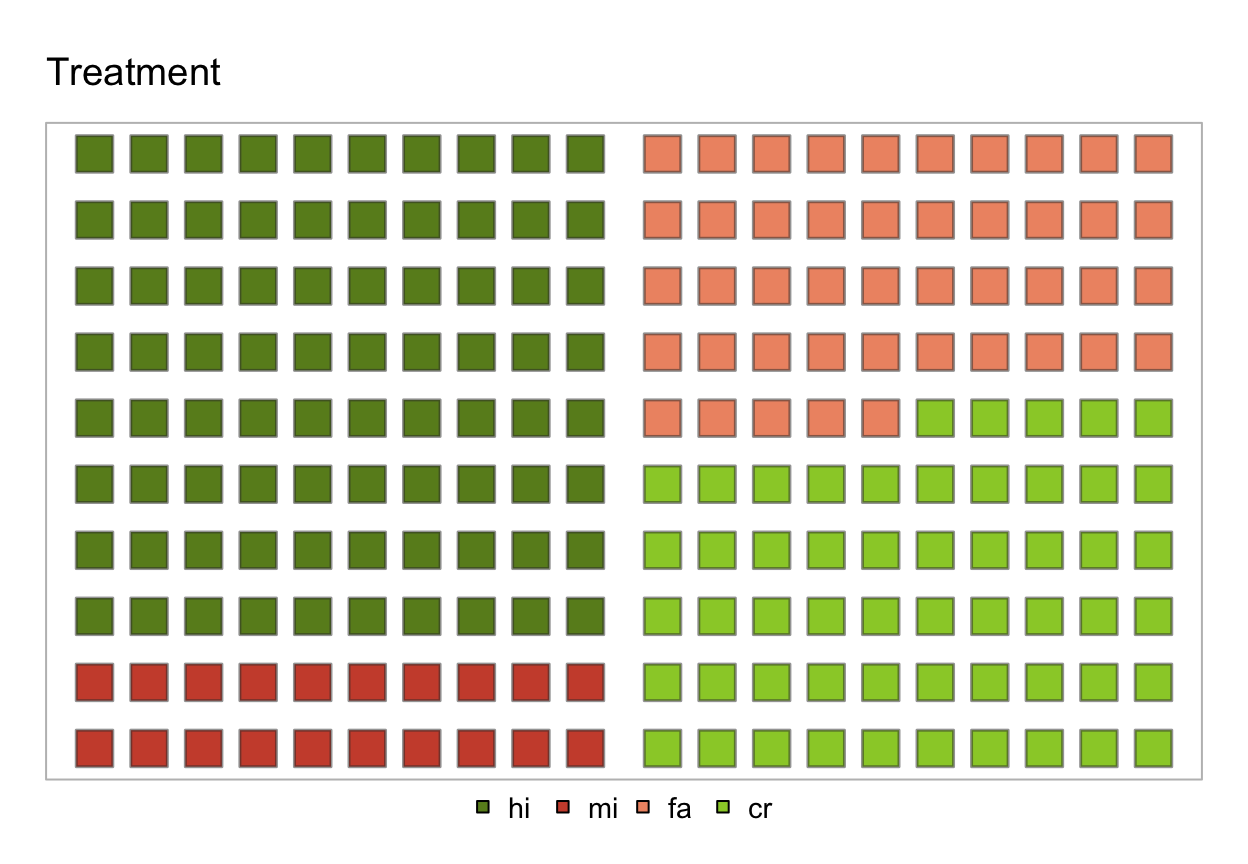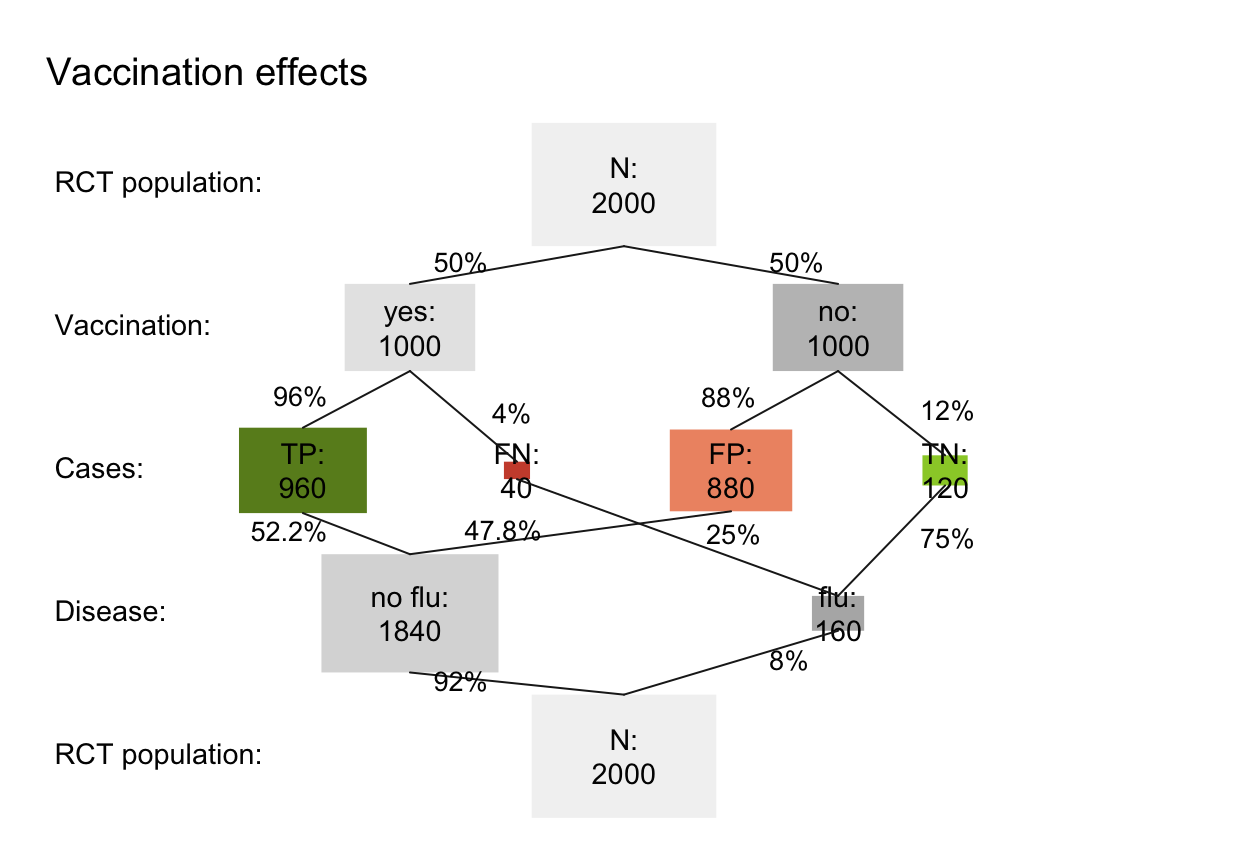read_popu interprets a data frame df (that contains individual observations of some population) and returns a scenario of class "riskyr".

read_popu(df = popu, ix_by_top = 1, ix_by_bot = 2, ix_sdt = 3,
hi_lbl = txt$hi_lbl, mi_lbl = txt$mi_lbl, fa_lbl = txt$fa_lbl, cr_lbl = txt$cr_lbl, ...)

## Arguments

df A data frame providing a population popu of individuals, which are identified on at least 2 binary variables and classified into 4 cases in a 3rd variable. Default: df = popu (as data frame). Index of variable (column) providing the 1st (top) perspective (in df). Default: ix_by_top = 1 (1st column). Index of variable (column) providing the 2nd (bot) perspective (in df). Default: ix_by_bot = 2 (2nd column). Index of variable (column) providing a classification into 4 cases (in df). Default: ix_by_bot = 3 (3rd column). Variable label of cases classified as hi (TP). Variable label of cases classified as mi (FN). Variable label of cases classified as fa (FP). Variable label of cases classified as cr (TN). Additional parameters (to be passed to riskyr function).

## Value

An object of class "riskyr" describing a risk-related scenario.

## Details

Note that df needs to be structured according to the popu created by comp_popu.

the corresponding data frame popu; the corresponding generating function comp_popu; riskyr initializes a riskyr scenario.

Other riskyr scenario functions: plot.riskyr, riskyr, summary.riskyr

## Examples

# Generating and interpreting different scenario types:

# (A) Diagnostic/screening scenario (using default labels): ------
popu_diag <- comp_popu(hi = 4, mi = 1, fa = 2, cr = 3)
# popu_diag
scen_diag <- read_popu(popu_diag, scen_lbl = "Diagnostics", popu_lbl = "Population tested")
plot(scen_diag, type = "prism", area = "no", f_lbl = "namnum")# (B) Intervention/treatment scenario: ------
popu_treat <- comp_popu(hi = 80, mi = 20, fa = 45, cr = 55,
cond_lbl = "Treatment", cond_true_lbl = "pill", cond_false_lbl = "placebo",
dec_lbl = "Health status", dec_pos_lbl = "healthy", dec_neg_lbl = "sick")
# popu_treat
scen_treat <- read_popu(popu_treat, scen_lbl = "Treatment", popu_lbl = "Population treated")
plot(scen_treat, type = "prism", area = "sq", f_lbl = "namnum", p_lbl = "num")plot(scen_treat, type = "icon", lbl_txt = txt_org, col_pal = pal_org)# (C) Prevention scenario (e.g., vaccination): ------
popu_vacc <- comp_popu(hi = 960, mi = 40, fa = 880, cr = 120,
cond_lbl = "Vaccination", cond_true_lbl = "yes", cond_false_lbl = "no",
dec_lbl = "Disease", dec_pos_lbl = "no flu", dec_neg_lbl = "flu")
# popu_vacc
scen_vacc <- read_popu(popu_vacc, scen_lbl = "Prevention", popu_lbl = "Population vaccinated")
plot(scen_vacc, type = "prism", area = "sq", f_lbl = "namnum", col_pal = pal_bw, p_lbl = "num")